Publicité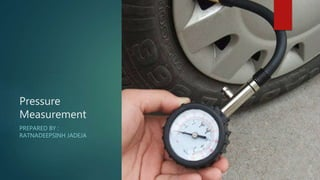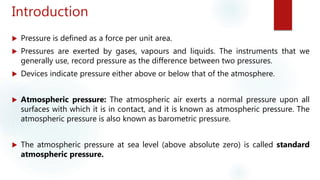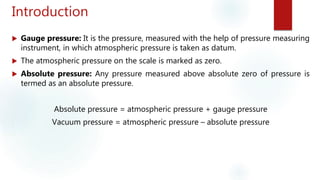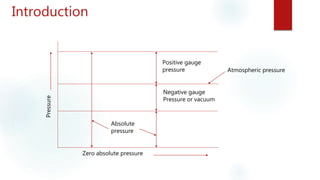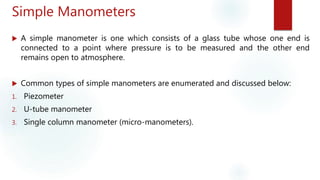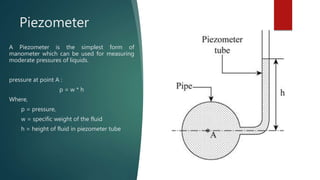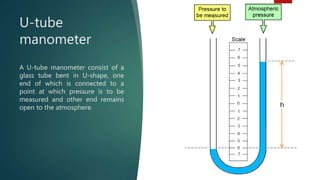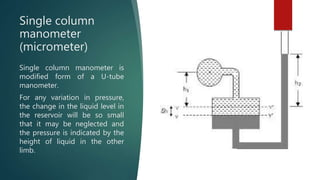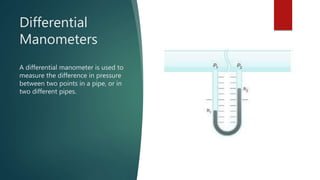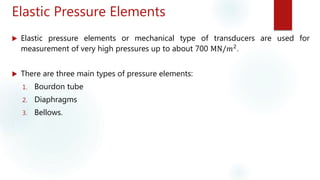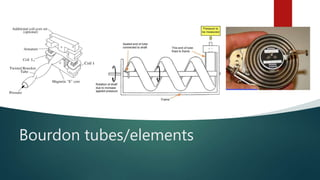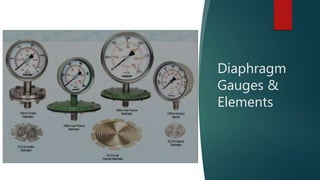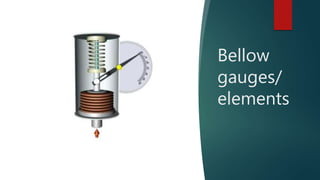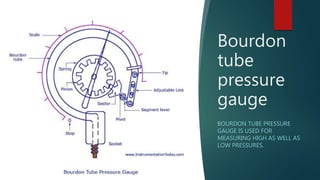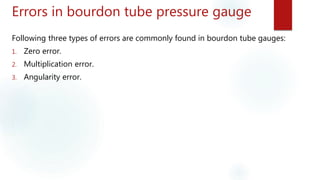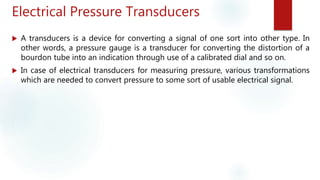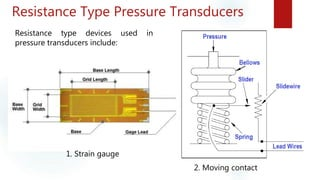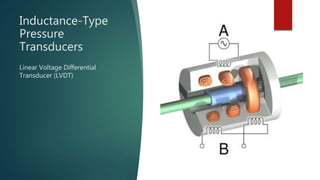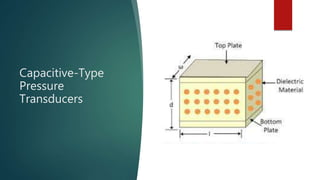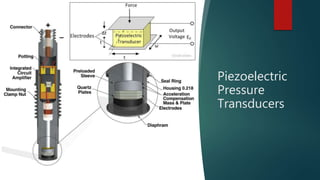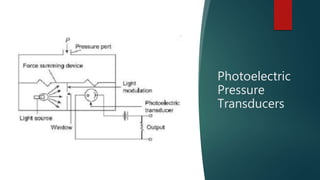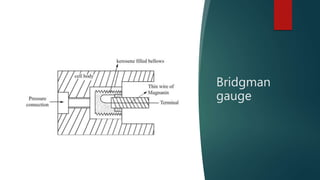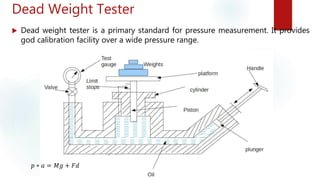1 sur 24
Publicité

### Pressure measurement

2. Introduction  Pressure is defined as a force per unit area.  Pressures are exerted by gases, vapours and liquids. The instruments that we generally use, record pressure as the difference between two pressures.  Devices indicate pressure either above or below that of the atmosphere.  Atmospheric pressure: The atmospheric air exerts a normal pressure upon all surfaces with which it is in contact, and it is known as atmospheric pressure. The atmospheric pressure is also known as barometric pressure.  The atmospheric pressure at sea level (above absolute zero) is called standard atmospheric pressure.
3. Introduction  Gauge pressure: It is the pressure, measured with the help of pressure measuring instrument, in which atmospheric pressure is taken as datum.  The atmospheric pressure on the scale is marked as zero.  Absolute pressure: Any pressure measured above absolute zero of pressure is termed as an absolute pressure. Absolute pressure = atmospheric pressure + gauge pressure Vacuum pressure = atmospheric pressure – absolute pressure
4. Introduction Positive gauge pressure Atmospheric pressure Negative gauge Pressure or vacuum Absolute pressure Pressure Zero absolute pressure
5. Simple Manometers  A simple manometer is one which consists of a glass tube whose one end is connected to a point where pressure is to be measured and the other end remains open to atmosphere.  Common types of simple manometers are enumerated and discussed below: 1. Piezometer 2. U-tube manometer 3. Single column manometer (micro-manometers).
6. Piezometer A Piezometer is the simplest form of manometer which can be used for measuring moderate pressures of liquids. pressure at point A : p = w * h Where, p = pressure, w = specific weight of the fluid h = height of fluid in piezometer tube
7. U-tube manometer A U-tube manometer consist of a glass tube bent in U-shape, one end of which is connected to a point at which pressure is to be measured and other end remains open to the atmosphere.
8. Single column manometer (micrometer) Single column manometer is modified form of a U-tube manometer. For any variation in pressure, the change in the liquid level in the reservoir will be so small that it may be neglected and the pressure is indicated by the height of liquid in the other limb.
9. Differential Manometers A differential manometer is used to measure the difference in pressure between two points in a pipe, or in two different pipes.
10. Elastic Pressure Elements  Elastic pressure elements or mechanical type of transducers are used for measurement of very high pressures up to about 700 MN/𝑚2 .  There are three main types of pressure elements: 1. Bourdon tube 2. Diaphragms 3. Bellows.
11. Bourdon tubes/elements
12. Diaphragm Gauges & Elements
13. Bellow gauges/ elements
14. Bourdon tube pressure gauge BOURDON TUBE PRESSURE GAUGE IS USED FOR MEASURING HIGH AS WELL AS LOW PRESSURES.
15. Errors in bourdon tube pressure gauge Following three types of errors are commonly found in bourdon tube gauges: 1. Zero error. 2. Multiplication error. 3. Angularity error.
16. Electrical Pressure Transducers  A transducers is a device for converting a signal of one sort into other type. In other words, a pressure gauge is a transducer for converting the distortion of a bourdon tube into an indication through use of a calibrated dial and so on.  In case of electrical transducers for measuring pressure, various transformations which are needed to convert pressure to some sort of usable electrical signal.
17. Resistance Type Pressure Transducers Resistance type devices used in pressure transducers include: 1. Strain gauge 2. Moving contact
18. Inductance-Type Pressure Transducers Linear Voltage Differential Transducer (LVDT)
19. Reluctance-Type Pressure Transducers
20. Capacitive-Type Pressure Transducers
21. Piezoelectric Pressure Transducers
22. Photoelectric Pressure Transducers
23. Bridgman gauge
24. Dead Weight Tester  Dead weight tester is a primary standard for pressure measurement. It provides god calibration facility over a wide pressure range. 𝑝 ∗ 𝑎 = 𝑀𝑔 + 𝐹𝑑
Publicité